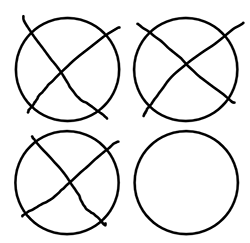Description: Draw pictures to represent math problems under 10.

Question 1 (Short Answer, 10 points)

What is 4-3=?

You can draw circles for the numbers to show how you got the answer.

Example below:Question 2 (Short Answer, 10 points)

What is 10-3=?

You can draw squares for the numbers to show how you got the answer.

Question 3 (Short Answer, 10 points)

What is 2+0=?

You can draw objects or stars for the numbers to show how you got the answer.

Question 4 (Short Answer, 10 points)

What is 8-2=?

Draw objects or triangles for the numbers to show how you got the answer.

Question 5 (Short Answer, 10 points)

What is 4+3=?